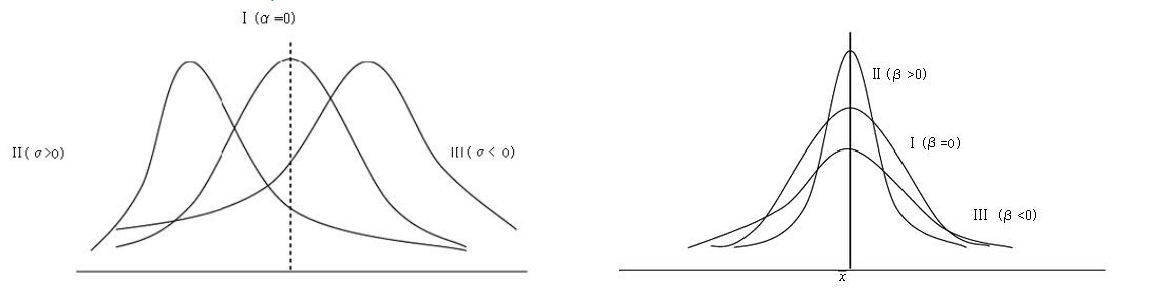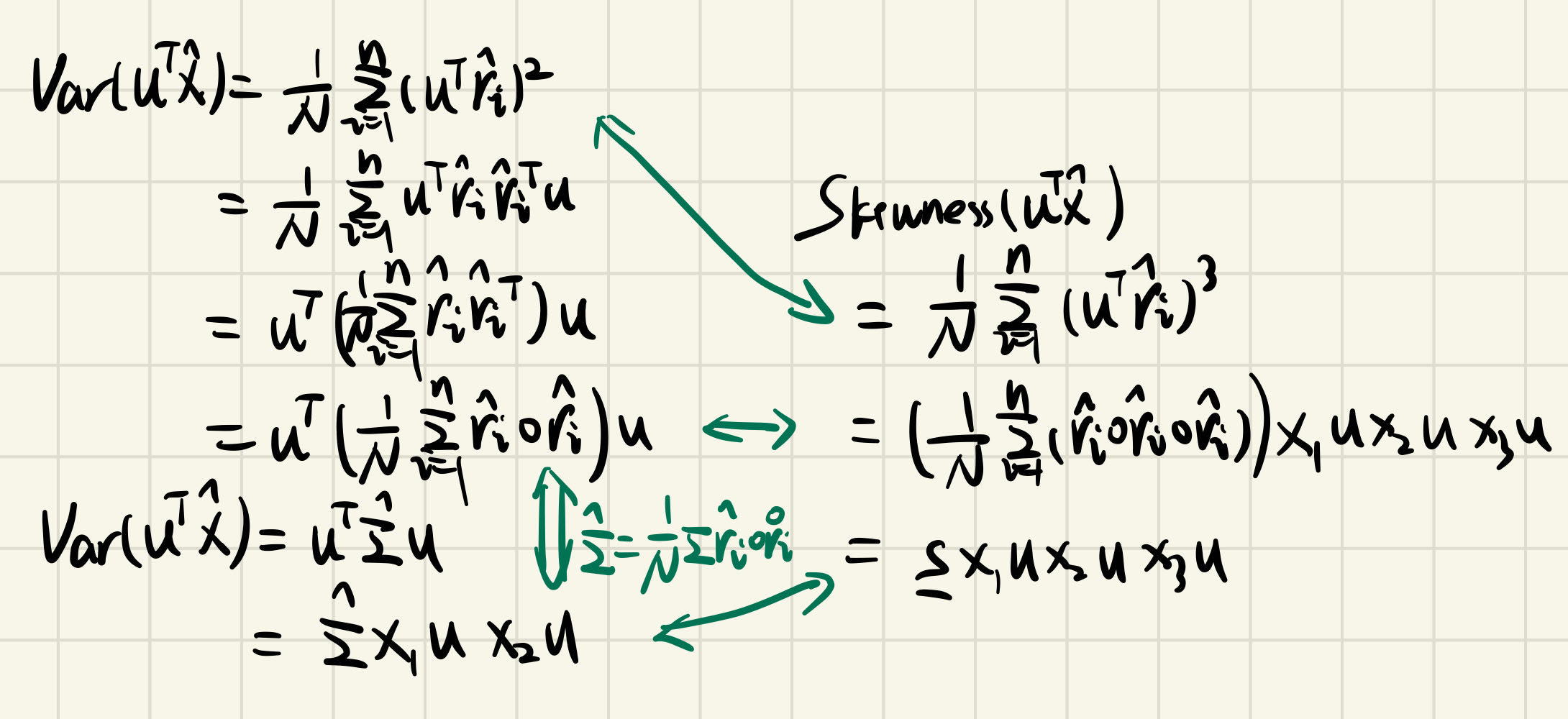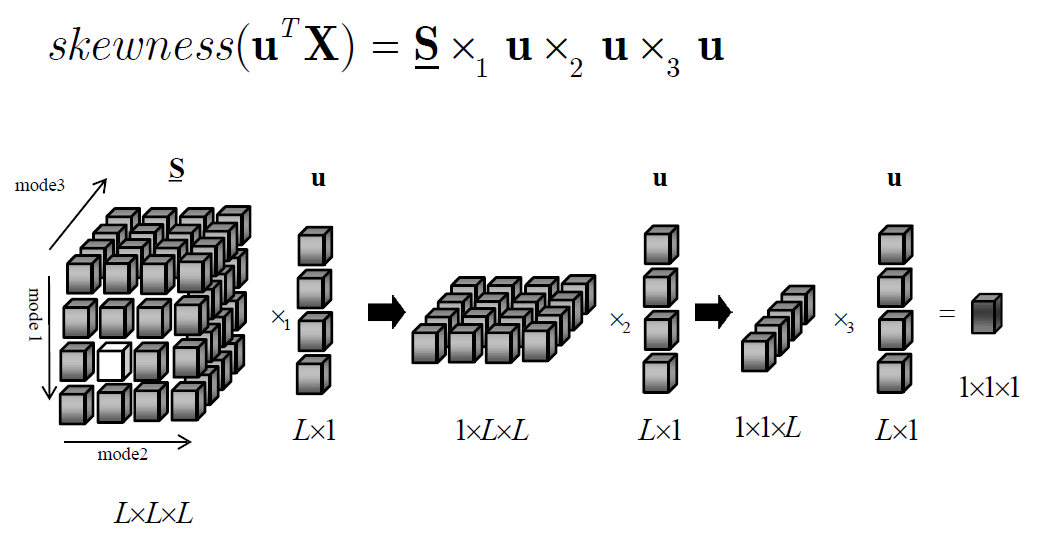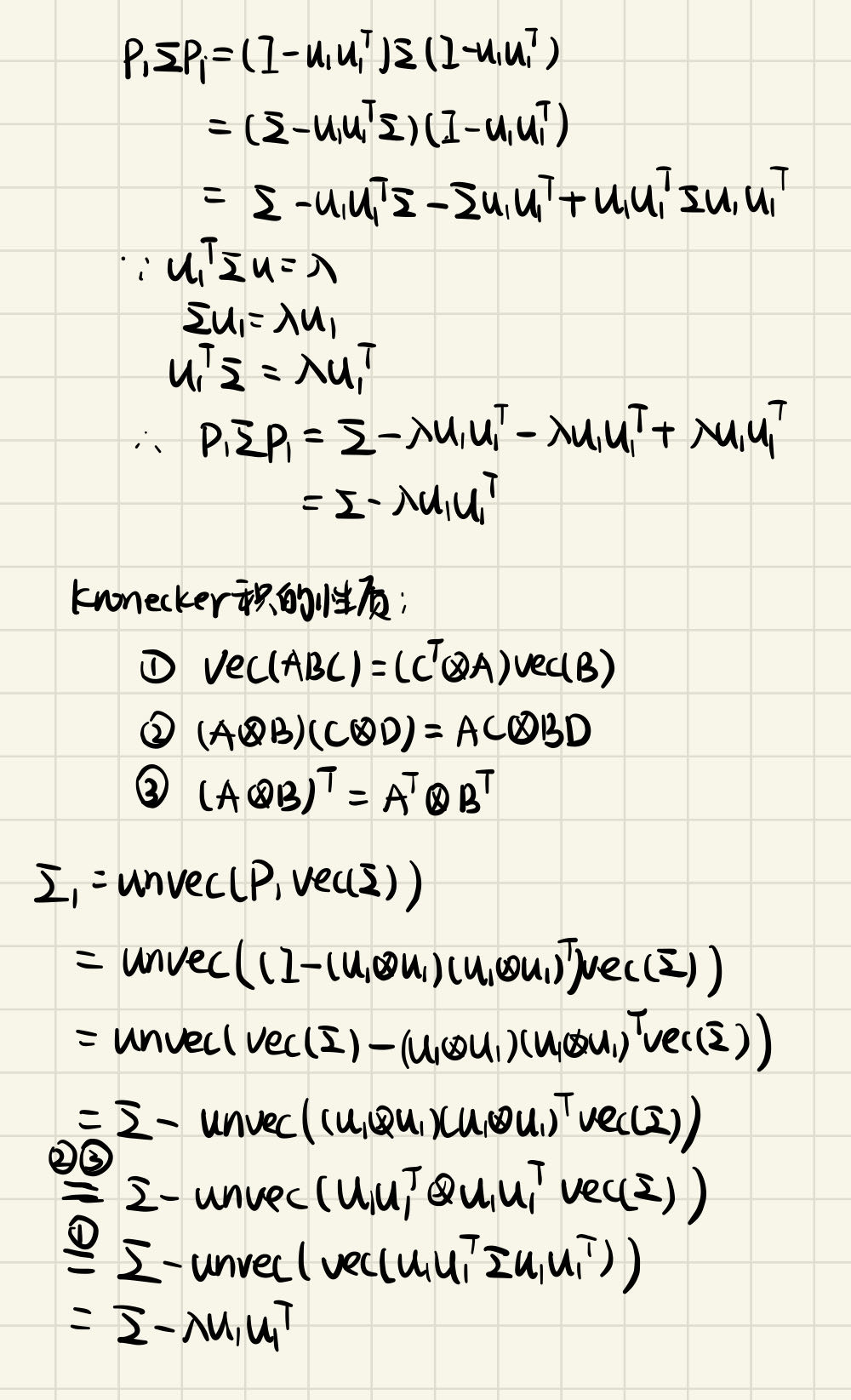## 问题背景

$$\begin{matrix} \mathbf{X_1}=a_{11}\mathbf{s_1}+a_{12}\mathbf{s_2}+a_{13}\mathbf{s_3} \\ \mathbf{X_2}=a_{21}\mathbf{s_1}+a_{22}\mathbf{s_2}+a_{23}\mathbf{s_3} \\ \mathbf{X_3}=a_{31}\mathbf{s_1}+a_{32}\mathbf{s_2}+a_{33}\mathbf{s_3} \end{matrix}$$

## 评价指标## 偏度和峭度计算方法

### 偏度

$$\operatorname{Skewness}(\mathbf{x})=\frac{E\left(x_i-\bar{x}\right)^3}{\sigma^3}=\frac{\frac{1}{n} \sum_{i=1}^n\left(x_i-\bar{x}\right)^3}{\operatorname{Var}(\mathbf{x})^{3 / 2}}$$

### 峭度

$$\operatorname{Kurtosis}(\mathbf{x})=\frac{E\left(x_i-\bar{x}\right)^4}{\sigma^4}-3=\frac{\frac{1}{n} \sum_{i=1}^n\left(x_i-\bar{x}\right)^4}{\operatorname{Var}(\mathbf{x})^2}-3$$

## 任意方向的偏度计算

### 分析

$$\operatorname{Skewness}(\mathbf{x})=\frac{E\left(x_i-\bar{x}\right)^3}{\sigma^3}=\frac{\frac{1}{n} \sum_{i=1}^n\left(x_i-\bar{x}\right)^3}{\operatorname{Var}(\mathbf{x})^{3 / 2}}$$

### 数据的白化

\begin{aligned} \hat{\mathbf{\Sigma}}&=\frac{1}{N}\hat{\mathbf{X}}\hat{\mathbf{X}}^{T}\\ &= \frac{1}{N}\mathbf{F}^T\mathbf{X}\mathbf{X}^{T}\mathbf{F} \end{aligned}

\begin{aligned} \hat{\mathbf{\Sigma}}&=\frac{1}{N}\hat{\mathbf{X}}\hat{\mathbf{X}}^{T}\\ &= \frac{1}{N}\mathbf{F}^T\mathbf{X}\mathbf{X}^{T}\mathbf{F}\\ &= \mathbf{F}^T\mathbf{U}\mathbf{D}\mathbf{U}^{T}\mathbf{F}\\ &= \mathbf{F}^T\mathbf{U}\mathbf{D}^{\frac{1}{2}}\mathbf{D}^{\frac{1}{2}}\mathbf{U}^{T}\mathbf{F}\\ &= \mathbf{F}^T(\mathbf{U}\mathbf{D}^{\frac{1}{2}})(\mathbf{D}^{\frac{1}{2}}\mathbf{U}^{T})\mathbf{F}\\ &= \mathbf{I} \end{aligned}

$$\hat{\mathbf{X}}=\mathbf{F}^{\mathrm{T}} \mathbf{X}=\left[\begin{array}{c} \hat{X}_1 \\ \vdots \\ \hat{X}_L \end{array}\right]=\left[\hat{\mathbf{r}}_1, \hat{\mathbf{r}}_2, \cdots, \hat{\mathbf{r}}_N\right]$$

$$\mathbf{u}^{\mathrm{T}} \hat{\mathbf{X}}=\left[\mathbf{u}^{\mathrm{T}} \hat{\mathbf{r}}_1, \mathbf{u}^{\mathrm{T}} \hat{\mathbf{r}}_2, \cdots, \mathbf{u}^{\mathrm{T}} \hat{\mathbf{r}}_N\right]$$

### 协偏度张量

\begin{aligned} \text { Skewness }\left(\mathbf{u}^{\mathrm{T}} \hat{\mathbf{X}}\right) &= \operatorname{Skewness}\left(\left[\mathbf{u}^{\mathrm{T}} \hat{\mathbf{r}}_1, \mathbf{u}^{\mathrm{T}} \hat{\mathbf{r}}_2, \cdots, \mathbf{u}^{\mathrm{T}} \hat{\mathbf{r}}_N\right]\right) \\ &= \frac{\frac{1}{N} \sum_{i=1}^N\left(\mathbf{u}^{\mathrm{T}} \hat{\mathbf{r}}_i-0\right)^3}{\operatorname{Var}\left(\mathbf{u}^{\mathrm{T}} \hat{\mathbf{X}}\right)^{3 / 2}} \\ &= \frac{1}{N} \sum_{i=1}^N\left(\mathbf{u}^{\mathrm{T}} \hat{\mathbf{r}}_i\right)^3 \\ &=\left(\frac{1}{N} \sum_{i=1}^N\left(\hat{\mathbf{r}}_i \circ \hat{\mathbf{r}}_i \circ \hat{\mathbf{r}}_i\right)\right)\times_1 \mathbf{u} \times_2 \mathbf{u} \times_3 \mathbf{u} \\ &= \underline{\mathbf{S}} \times_1 \mathbf{u} \times_2 \mathbf{u} \times_3 \mathbf{u} \end{aligned}### 协偏度张量的计算

#### 外积法（列向量角度）

$$\underline{\mathbf{S}}=\frac{1}{N} \sum_{i=1}^N \hat{\mathbf{r}}_i^3=\frac{1}{N} \sum_{i=1}^N \hat{\mathbf{r}}_i \circ \hat{\mathbf{r}}_i \circ \hat{\mathbf{r}}_i$$

#### 内积法（行向量角度）

\begin{aligned} & s_{i j k}=\frac{1}{N} \sum_{l=1}^N \hat{X}_i(l) \hat{X}_j(l) \hat{X}_k(l) \\ & s_{i j k}=s_{i k j}=s_{j i k}=s_{j k i}=s_{k i j}=s_{k j i} \end{aligned}

## 主偏度分析

\begin{aligned} & \max g(\mathbf{u})=\underline{\mathbf{S}} \times_1 \mathbf{u} \times_2 \mathbf{u} \times_3 \mathbf{u} \\ & \text { s.t. } \mathbf{u}^T \mathbf{u}=1 \end{aligned}

$$\underline{\mathbf{S}} \times_1 \mathbf{u} \times_3 \mathbf{u}=\lambda \mathbf{u}$$

\begin{aligned} \mathbf{u} & =\underline{\mathbf{S}} \times_1 \mathbf{u} \times_3 \mathbf{u} \\ \mathbf{u} & =\frac{\mathbf{u}}{|\mathbf{u}|} \end{aligned}

### 正交投影法

\begin{aligned} & \mathbf{P}_1=\mathbf{I}-\mathbf{u}_1 \mathbf{u}_1^{\#}, \quad \mathbf{X}_1=\mathbf{P}_1 \hat{\mathbf{X}} \\ & \underline{\mathbf{S}}_1=\frac{1}{N} \sum_{i=1}^N\left(\mathbf{P}_1 \hat{\mathbf{r}_i}\right)^3=\frac{1}{N} \sum_{i=1}^N \mathbf{P}_1 \hat{\mathbf{r}_i} \circ \mathbf{P}_1 \hat{\mathbf{r}_i} \circ \mathbf{P}_1 \hat{\mathbf{r}_i} \\ & =\underline{\mathbf{S}} \times_1 \mathbf{P}_1 \times_2 \mathbf{P}_1 \times_3 \mathbf{P}_1 \\ & \mathbf{u}=\underline{\mathbf{S}}_1 \times_1 \mathbf{u} \times_3 \mathbf{u} \end{aligned}

### Deflation 法

\begin{aligned} & \underline{\mathbf{S}}_1=\underline{\mathbf{S}}-\lambda \mathbf{u}_1 \circ \mathbf{u}_1 \circ \mathbf{u}_1 \\ & \mathbf{u}=\underline{\mathbf{S}}_1 \times_1 \mathbf{u} \times_3 \mathbf{u} \end{aligned}

### Kronecker 法

\begin{aligned} & \mathbf{P}_1=\mathbf{I}-\left(\mathbf{u}_1 \otimes \mathbf{u}_1 \otimes \mathbf{u}_1\right)\left(\mathbf{u}_1 \otimes \mathbf{u}_1 \otimes \mathbf{u}_1\right)^{\#} \\ & \underline{\mathbf{S}}_1=\operatorname{tensor}\left(\mathbf{P}_1 \operatorname{vec}(\underline{\mathbf{S}})\right) \\ & \mathbf{u}=\underline{\mathbf{S}}_1 \times_1 \mathbf{u} \times_3 \mathbf{u} \end{aligned}

Deflation 法与 Kronecker 法等价，但是与正交投影法并不等价。

### 上述三种方法在主成分分析的形式

\begin{aligned} & \mathbf{P}_1=\mathbf{I}-\mathbf{u}_1 \mathbf{u}_1^{\#}, \quad \mathbf{X}_1=\mathbf{P}_1 \mathbf{X} \\ & \Sigma_1=\frac{1}{N} \mathbf{X}_1 \mathbf{X}_1^{\mathrm{T}}=\mathbf{P}_1 \Sigma \mathbf{P}_1 \\ & =\mathbf{\Sigma} \times_1 \mathbf{P}_1 \times_2 \mathbf{P}_1 \\ & \Sigma_1 \mathbf{u}=\lambda \mathbf{u} \end{aligned}

Deflation 法：

\begin{aligned} & \Sigma_1=\Sigma-\lambda \mathbf{u}_1 \mathbf{u}_1^{\mathrm{T}} \\ & \Sigma_1 \mathbf{u}=\lambda \mathbf{u} \end{aligned}

Kronecker 法：

\begin{aligned} & \mathbf{P}_1=\mathbf{I}-\left(\mathbf{u}_1 \otimes \mathbf{u}_1\right)\left(\mathbf{u}_1 \otimes \mathbf{u}_1\right)^{\#} \\ & \Sigma_1=\operatorname{unvec}\left(\mathbf{P}_1 \operatorname{vec}(\Sigma)\right) \\ & \Sigma_1 \mathbf{u}=\lambda \mathbf{u} \end{aligned}## 主偏度分析步骤

### 预处理

1. 数据中心化
2. 计算协方差矩阵：$\Sigma=\frac{1}{N}\mathbf{X}\mathbf{X}^T$
3. 计算协方差矩阵的特征值和特征向量：$\Sigma \mathbf{U}=\mathbf{U} \Lambda$
4. 计算白化算子：$\mathbf{F}=\mathbf{U} \Lambda^{-1 / 2}$
5. 对数据进行白化：$\hat{\mathbf{X}}=\mathbf{F}^{\mathrm{T}} \mathbf{X}$
6. 计算协偏度张量：内积法/外积法

### 主偏度变换

1. 计算数据的第一极值偏度方向 $\mathbf{u_1}$：

\begin{aligned} \mathbf{u} & =\underline{\mathbf{S}} \times_1 \mathbf{u} \times_3 \mathbf{u} \\ \mathbf{u} & =\frac{\mathbf{u}}{|\mathbf{u}|} \end{aligned}

1. 利用正交投影法等消除第一个主偏度方向的影响
2. 计算新的协偏度张量：

$$\underline{\mathbf{S}}_1=\underline{\mathbf{S}} \times{ }_1 \mathbf{P}_1 \times{ }_2 \mathbf{P}_1 \times_3 \mathbf{P}_1$$

1. 再利用固定点法，求第二极值偏度方向

## PSA 几何解释

PCA 的几何结构是一个椭圆，PSA 的几何结构是一个类似于多个“花瓣”组成的图形，如下红色部分：## 评论

1.czar-alex
8月前
2023-4-06 21:33:22

博主，数学公式不正确显示是我的浏览器的问题吗，还是Argon主题选项里没兼容数学公式

•博主
czar-alex
8月前
2023-4-06 22:19:20

我刚测试了一下，我电脑和手机上的chrome浏览器是可以的，但是edge就没渲染，目前我还不知道问题出在了哪?

## 发送评论编辑评论

|´・ω・)ノ
ヾ(≧∇≦*)ゝ
(☆ω☆)
（╯‵□′）╯︵┴─┴
￣﹃￣
(/ω＼)
∠( ᐛ 」∠)＿
(๑•̀ㅁ•́ฅ)
→_→
୧(๑•̀⌄•́๑)૭
٩(ˊᗜˋ*)و
(ノ°ο°)ノ
(´இ皿இ｀)
⌇●﹏●⌇
(ฅ´ωฅ)
(╯°A°)╯︵○○○
φ(￣∇￣o)
ヾ(´･ ･｀｡)ノ"
( ง ᵒ̌皿ᵒ̌)ง⁼³₌₃
(ó﹏ò｡)
Σ(っ °Д °;)っ
( ,,´･ω･)ﾉ"(´っω･｀｡)
╮(╯▽╰)╭
o(*////▽////*)q
＞﹏＜
( ๑´•ω•) "(ㆆᴗㆆ)
( ゜- ゜)つロ
_(:з」∠)_
（⌒▽⌒）
（￣▽￣）
(=・ω・=)
(*°▽°*)八(*°▽°*)♪
✿ヽ(°▽°)ノ✿
(¦3【▓▓】
눈_눈
(ಡωಡ)
_(≧∇≦」∠)_
━━━∑(ﾟ□ﾟ*川━
(｀・ω・´)
(￣3￣)
✧(≖ ◡ ≖✿)
(･∀･)
(〜￣△￣)〜
→_→
(°∀°)ﾉ
╮(￣▽￣)╭
( ´_ゝ｀)
←_←
(;¬_¬)
(ﾟДﾟ≡ﾟдﾟ)!?
( ´･･)ﾉ(._.)
Σ(ﾟдﾟ;)
Σ( ￣□￣||)<
(´；ω；)
（/TДT)/
(^・ω・^)
(｡･ω･｡)
(●￣(ｴ)￣●)
ε=ε=(ノ≧∇≦)ノ
(´･_･)
(-_-#)
（￣へ￣）
(￣ε(#￣) Σ
(╯°口°)╯(┴—┴
ヽ(Д´)ﾉ
("▔□▔)/
(º﹃º )
(๑>؂<๑）
｡ﾟ(ﾟ´Д｀)ﾟ｡
(∂ω∂)
(┯_┯)
(・ω< )★
( ๑ˊ•̥▵•)੭₎₎
¥ㄟ(´･ᴗ･)ノ¥
Σ_(꒪ཀ꒪」∠)_
٩(๛ ˘ ³˘)۶❤
(๑‾᷅^‾᷅๑)

Emoji

tv_小电视